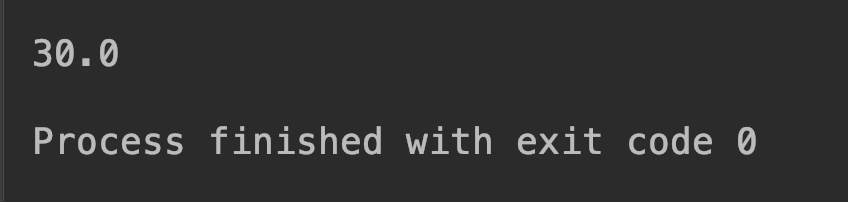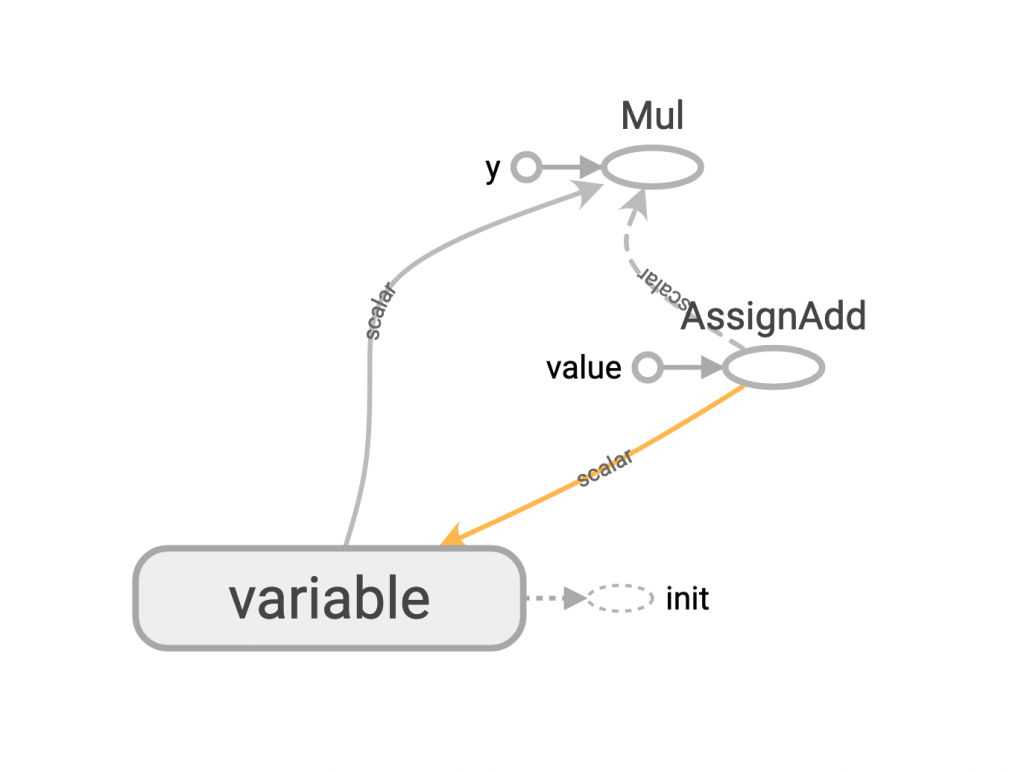DAY 15
0
Google Developers Machine Learning

【15】tensorflow 訓練技巧：control_dependencies 篇

with tf.control_dependencies(<要先被執行的op>):
<之後再執行的op>

v = tf.get_variable(name="variable", shape=(), initializer=tf.constant_initializer(0))

mul_op = tf.multiply(v, 10)

with tf.Session() as sess:
sess.run(tf.global_variables_initializer())
sess.run(tf.local_variables_initializer())

result = sess.run(mul_op)
print(result)github原始碼